Ex 5.1

Chapter 5 Class 10 Arithmetic Progressions
Serial order wise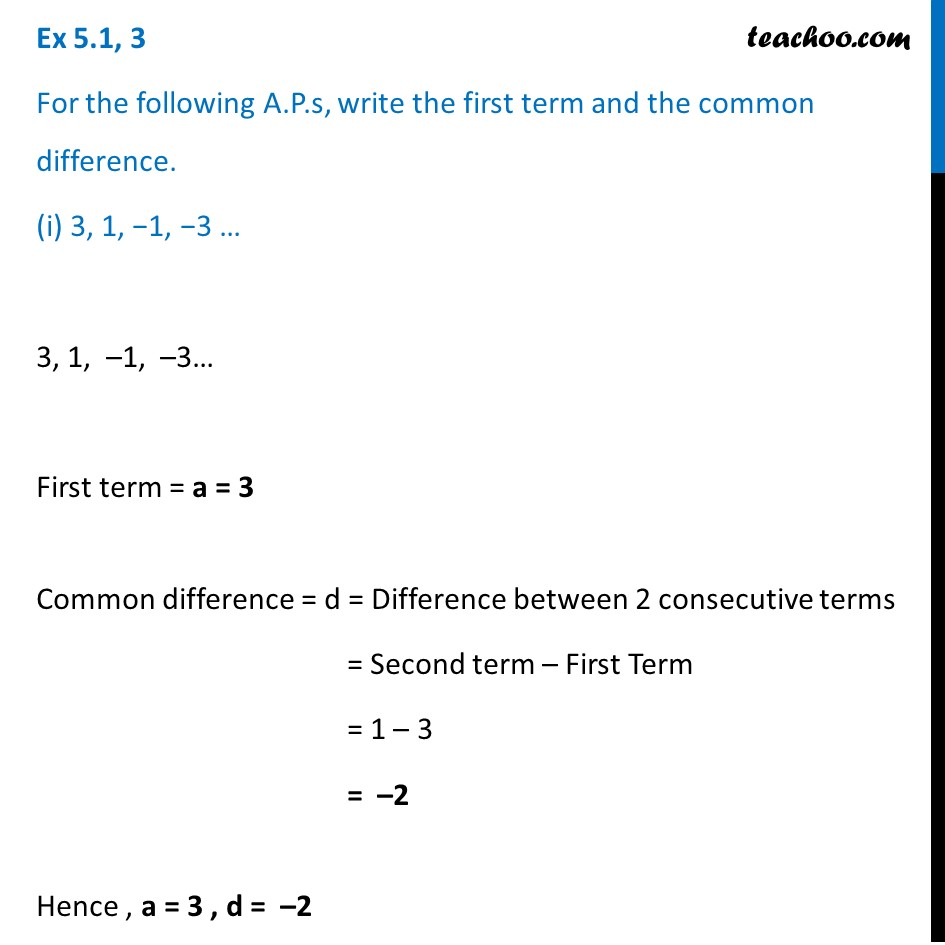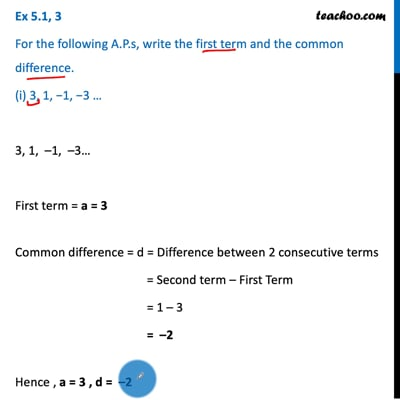This video is only available for Teachoo black users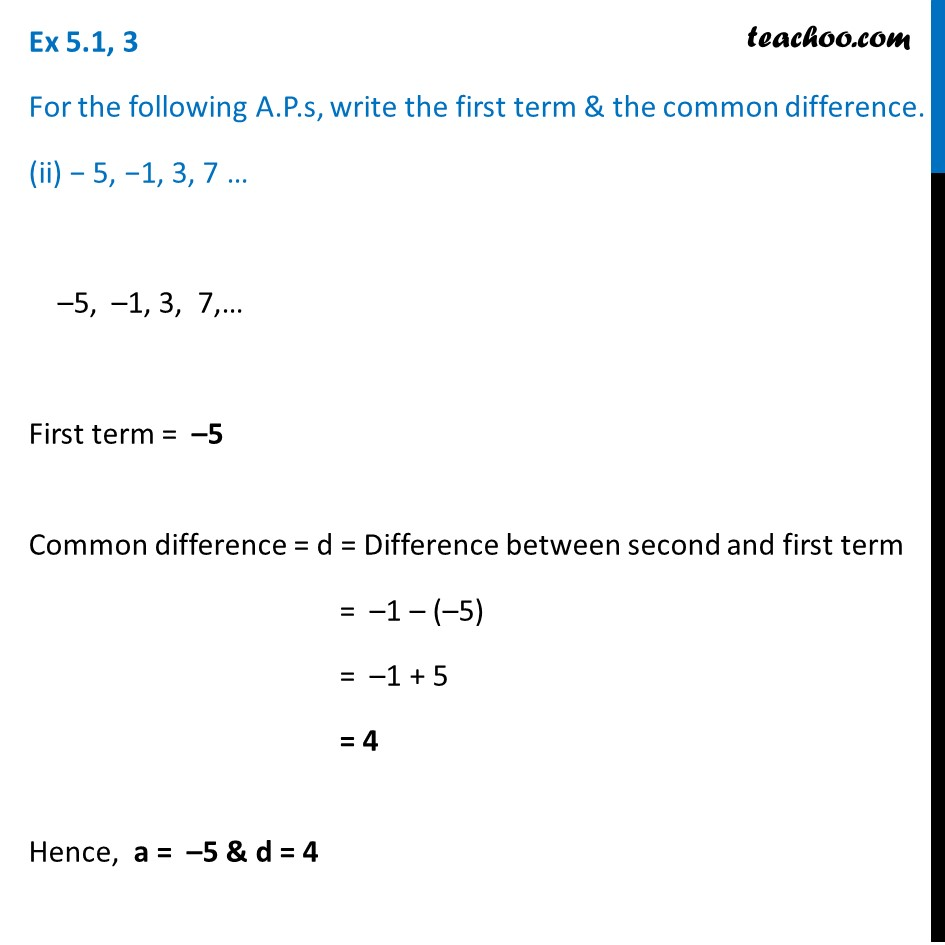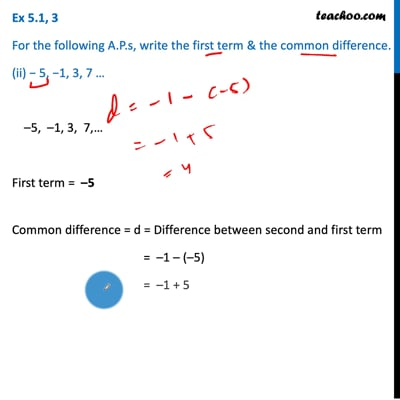This video is only available for Teachoo black users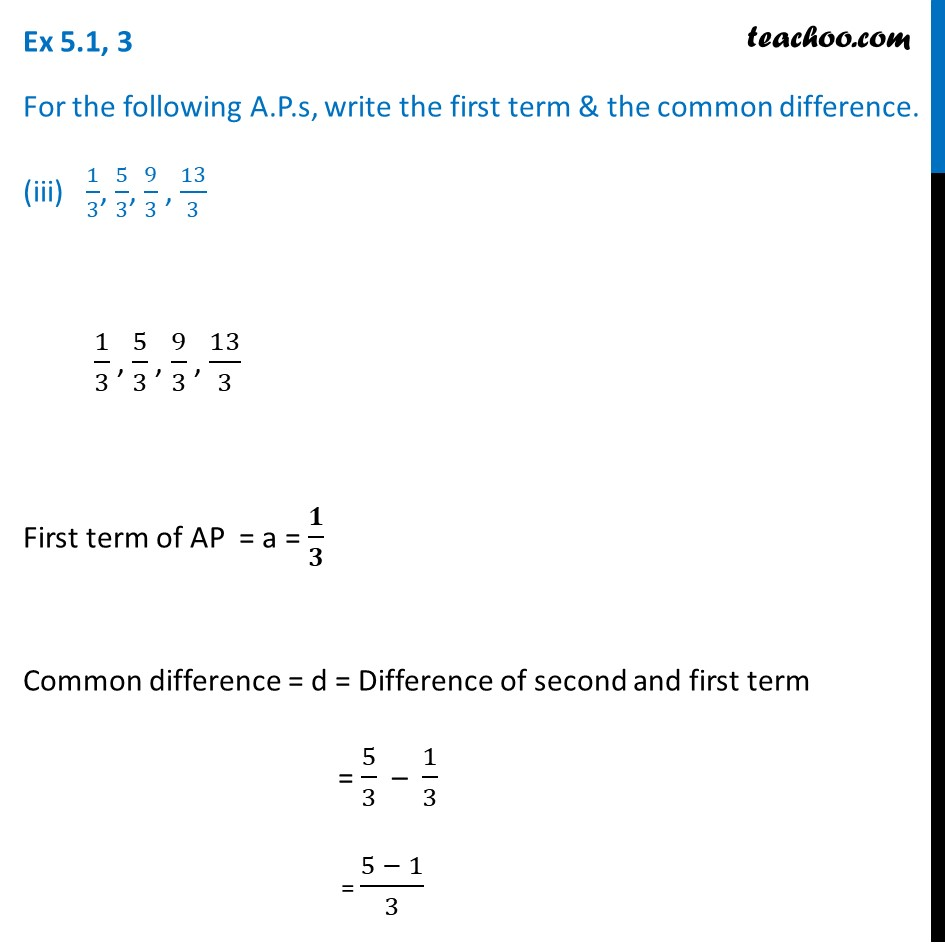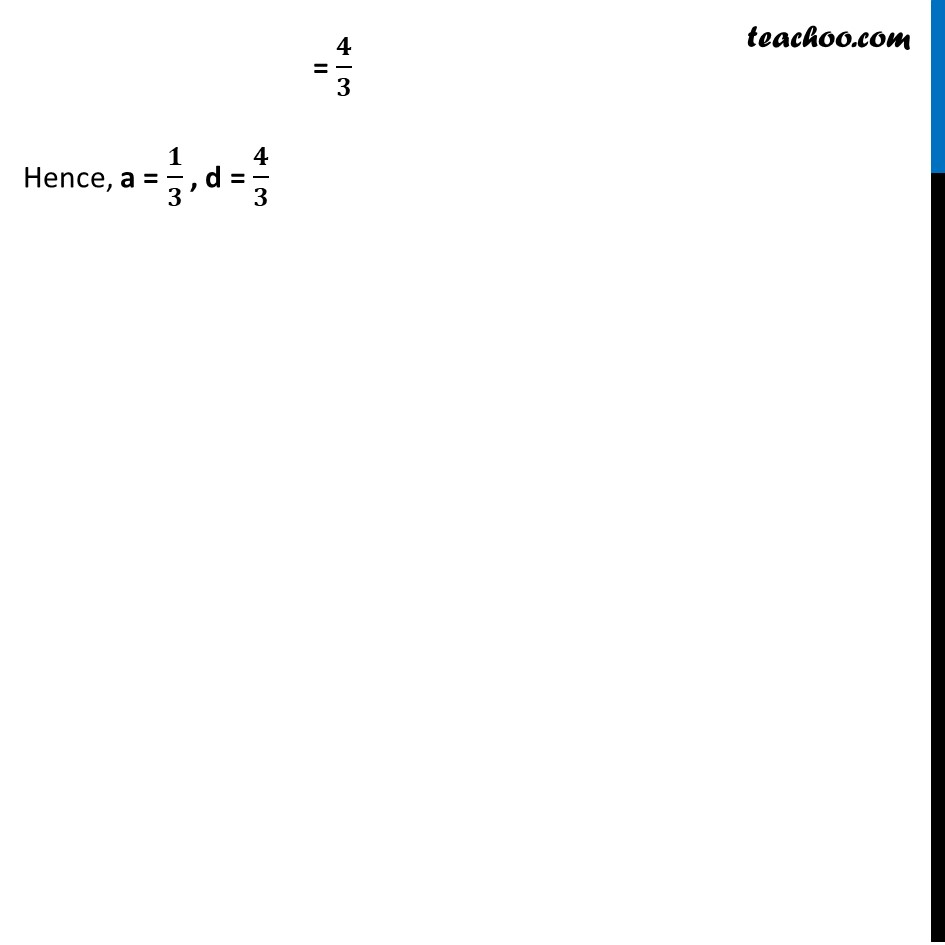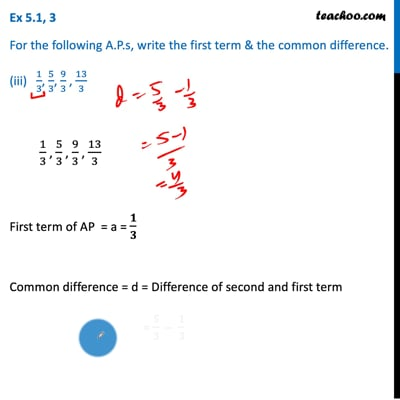This video is only available for Teachoo black users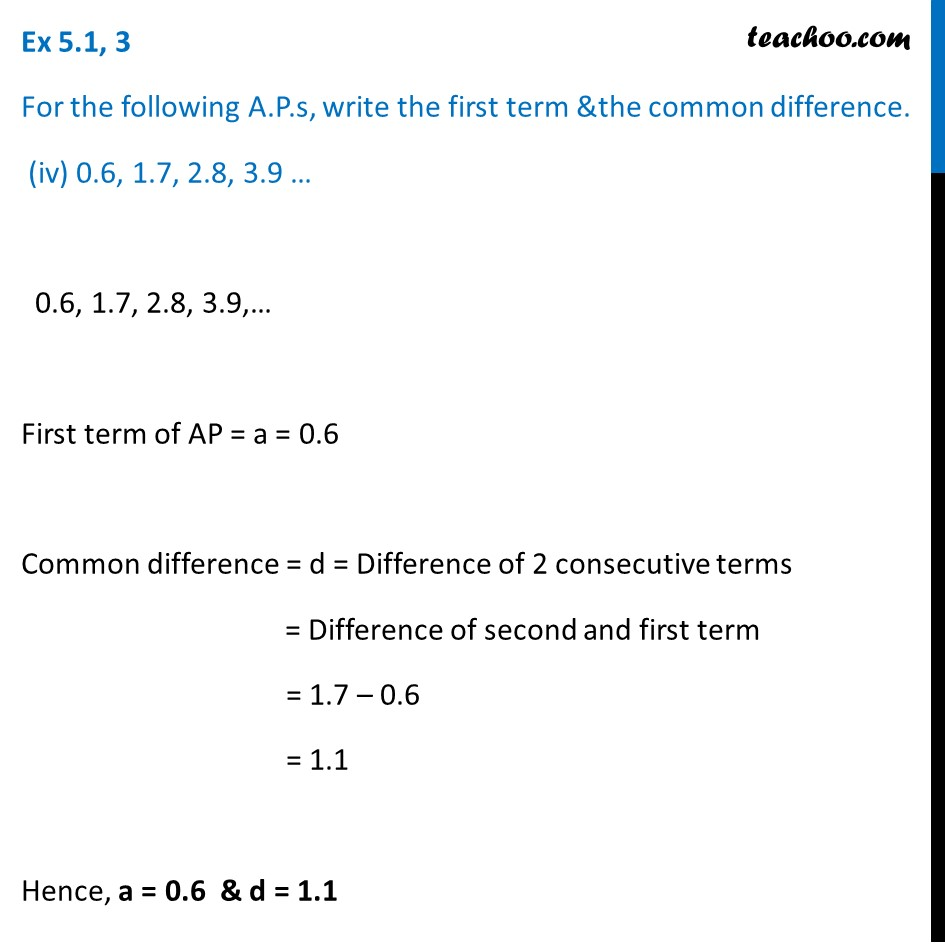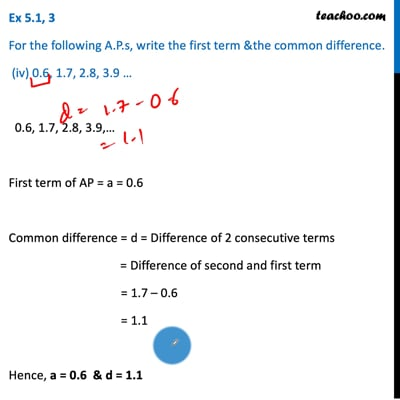This video is only available for Teachoo black users

Maths Crash Course - Live lectures + all videos + Real time Doubt solving!

### Transcript

Ex 5.1, 3 For the following A.P.s, write the first term and the common difference. (i) 3, 1, −1, −3 … 3, 1, –1, –3… First term = a = 3 Common difference = d = Difference between 2 consecutive terms = Second term – First Term = 1 – 3 = –2 Hence , a = 3 , d = –2 Ex 5.1, 3 For the following A.P.s, write the first term & the common difference. (ii) − 5, −1, 3, 7 … –5, –1, 3, 7,… First term = –5 Common difference = d = Difference between second and first term = –1 – (–5) = –1 + 5 = 4 Hence, a = –5 & d = 4 Ex 5.1, 3 For the following A.P.s, write the first term & the common difference. (iii) 1/3, 5/3, 9/3 , 13/3 1/3 , 5/3 , 9/3 , 13/3 First term of AP = a = 𝟏/𝟑 Common difference = d = Difference of second and first term = 5/3 – 1/3 = (5 − 1)/3 = 𝟒/𝟑 Hence, a = 𝟏/𝟑 , d = 𝟒/𝟑 Ex 5.1, 3 For the following A.P.s, write the first term &the common difference. (iv) 0.6, 1.7, 2.8, 3.9 … 0.6, 1.7, 2.8, 3.9,… First term of AP = a = 0.6 Common difference = d = Difference of 2 consecutive terms = Difference of second and first term = 1.7 – 0.6 = 1.1 Hence, a = 0.6 & d = 1.1